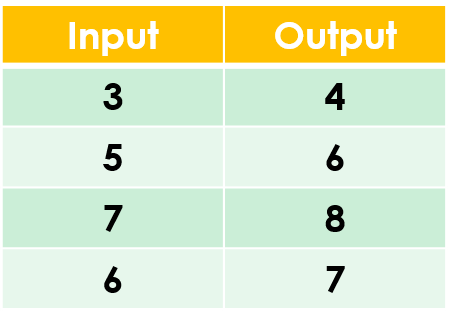1
visibility

Find the rule from the table below:• A

Add 3

• B

Add 1

The correct answer is "add 1".

Please Note:
Addition is the operation that increases a number by adding to it.

Solution:

To find the correct rule, we consider the given table.

We observe that the given rule makes each number increase. This implies addition.

We try adding 1 to each input number:

3 + 1 = 4
5 + 1 = 6
7 + 1 = 8
6 + 1 = 7

On adding 1 to the input numbers, we get the corresponding output numbers.

So, the rule is "add 1”.